# 442. Find All Duplicates in an Array 数组中重复的数据

• 作者： 负雪明烛
• id： fuxuemingzhu
• 个人博客： http://fuxuemingzhu.cn/open in new window
• 关键词：LeetCode，力扣，题解，算法，算法题，解析，C++, Java, Python，数组，重复数字，442

@TOC

## # 题目描述

输入：nums = [4,3,2,7,8,2,3,1]



输入：nums = [1,1,2]



输入：nums = 



1. n == nums.length
2. 1 <= n <= 10^5
3. 1 <= nums[i] <= n
4. nums 中的每个元素出现 一次 或 两次

## # 题目大意

• 数组长度是 ，每个数据的取值范围是
• 每个数字只出现一次或者两次
• 要求时间复杂度 ，空间复杂度是

## # 解题方法

### # 原地修改数组

• 数组长度是 ，每个数据的取值范围是

• 取反
• 增加偏移量

#### # 方法一：取反

• 从起始位置进行遍历，每次将下标的数字取反；
• 当遍历到值 为负数，需要忽略其负号。
• 若发现下标的数字已经是负数，说明之前出现过同样的数字 ，即找到了重复数字；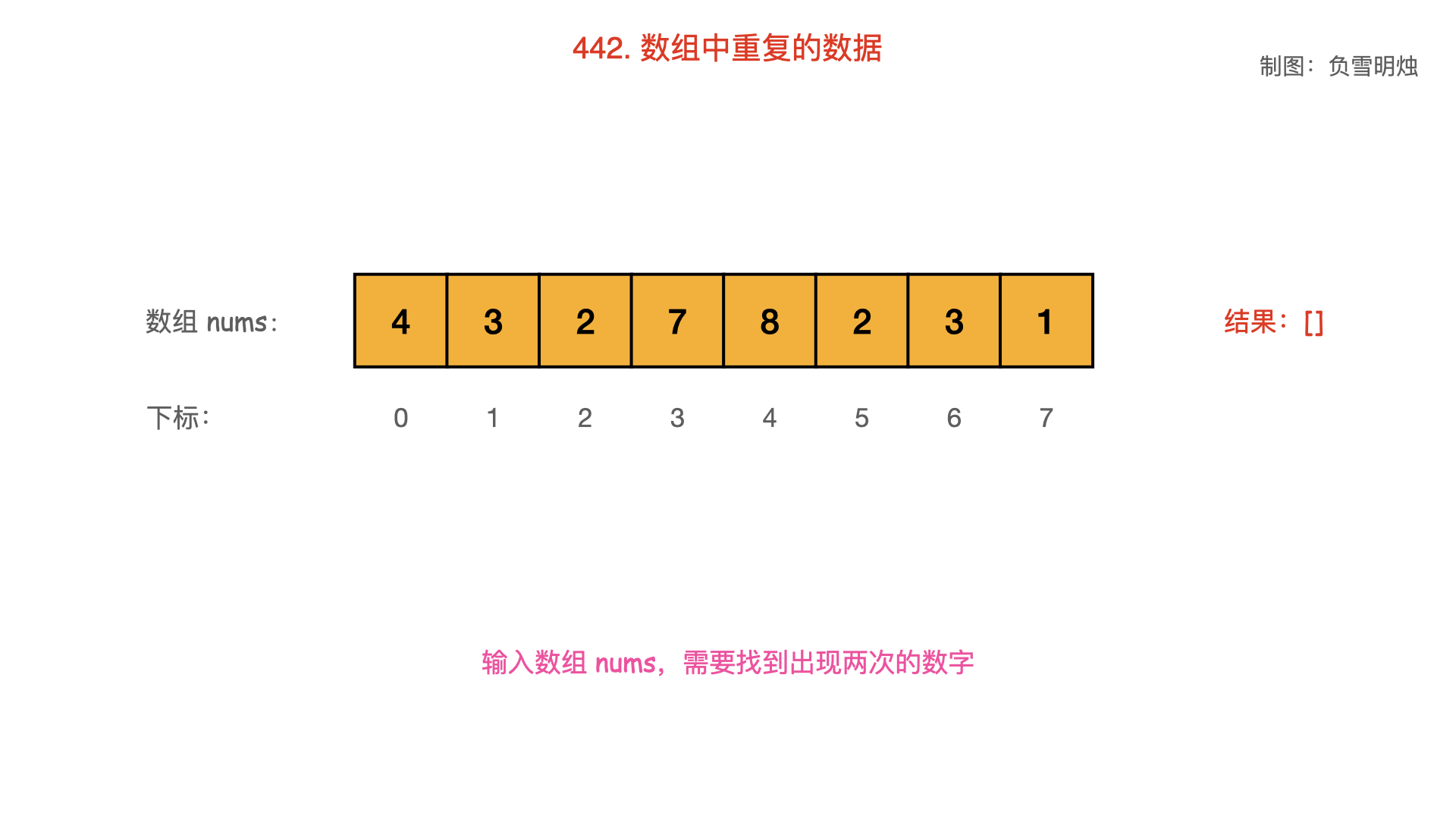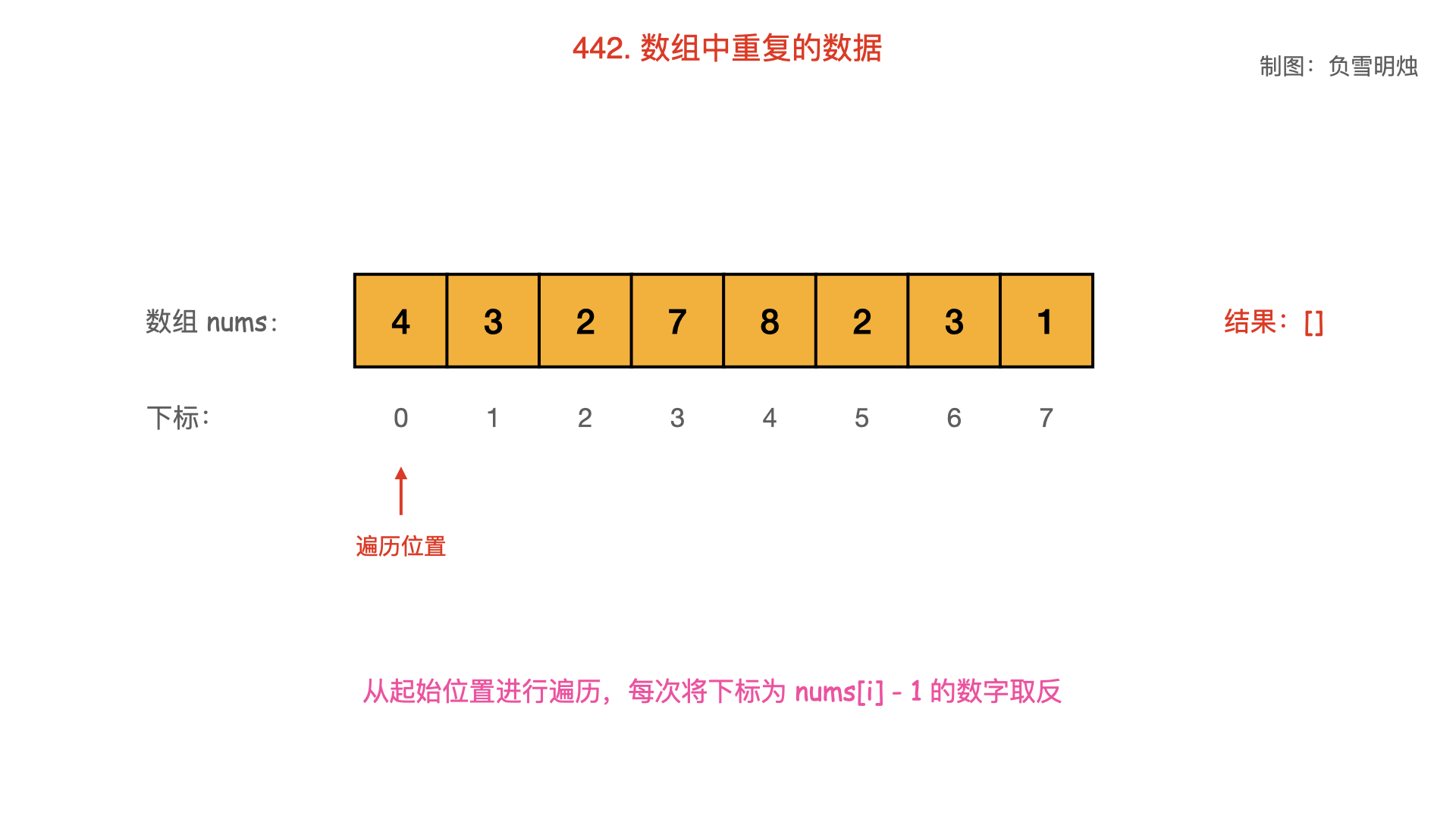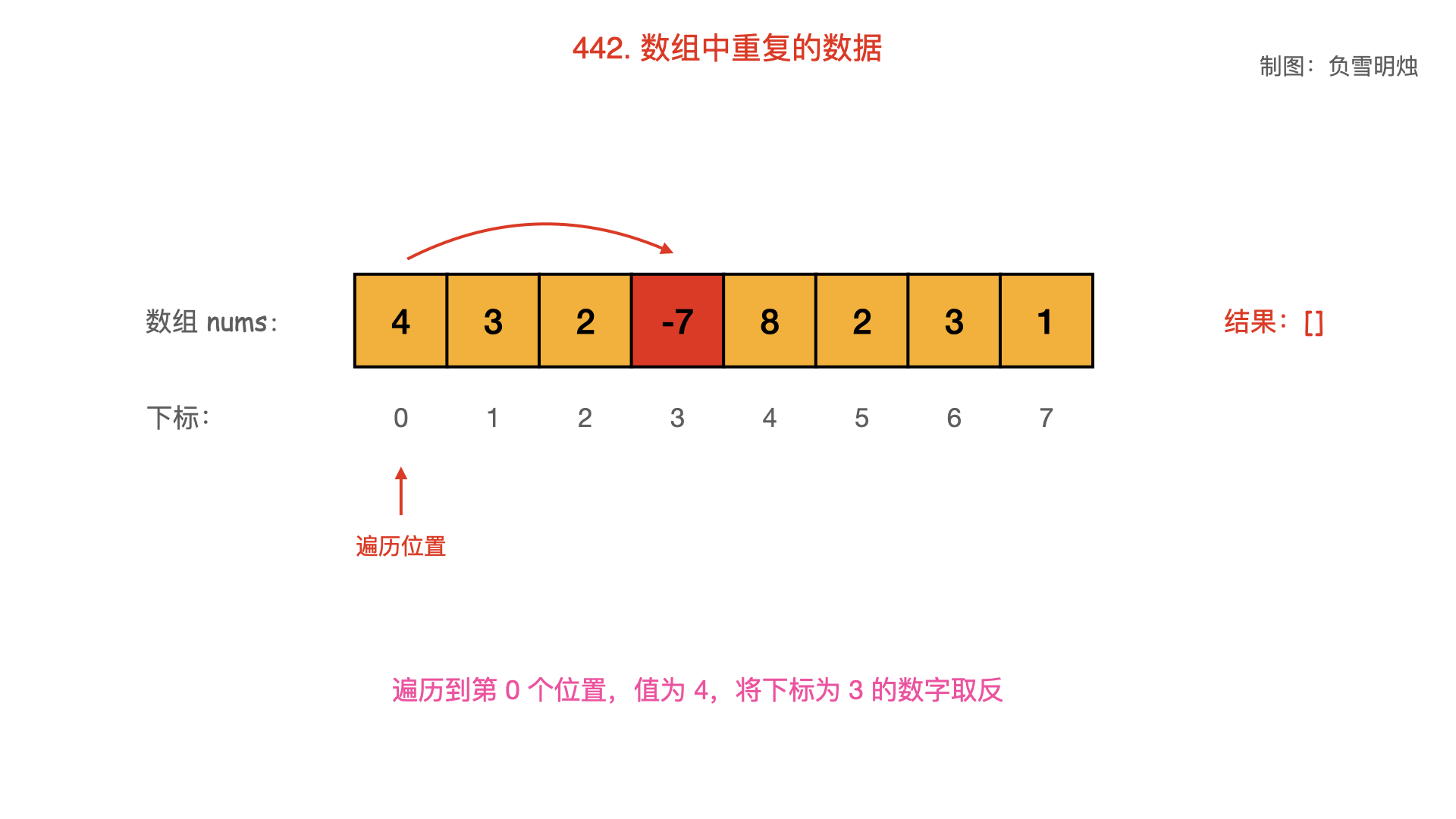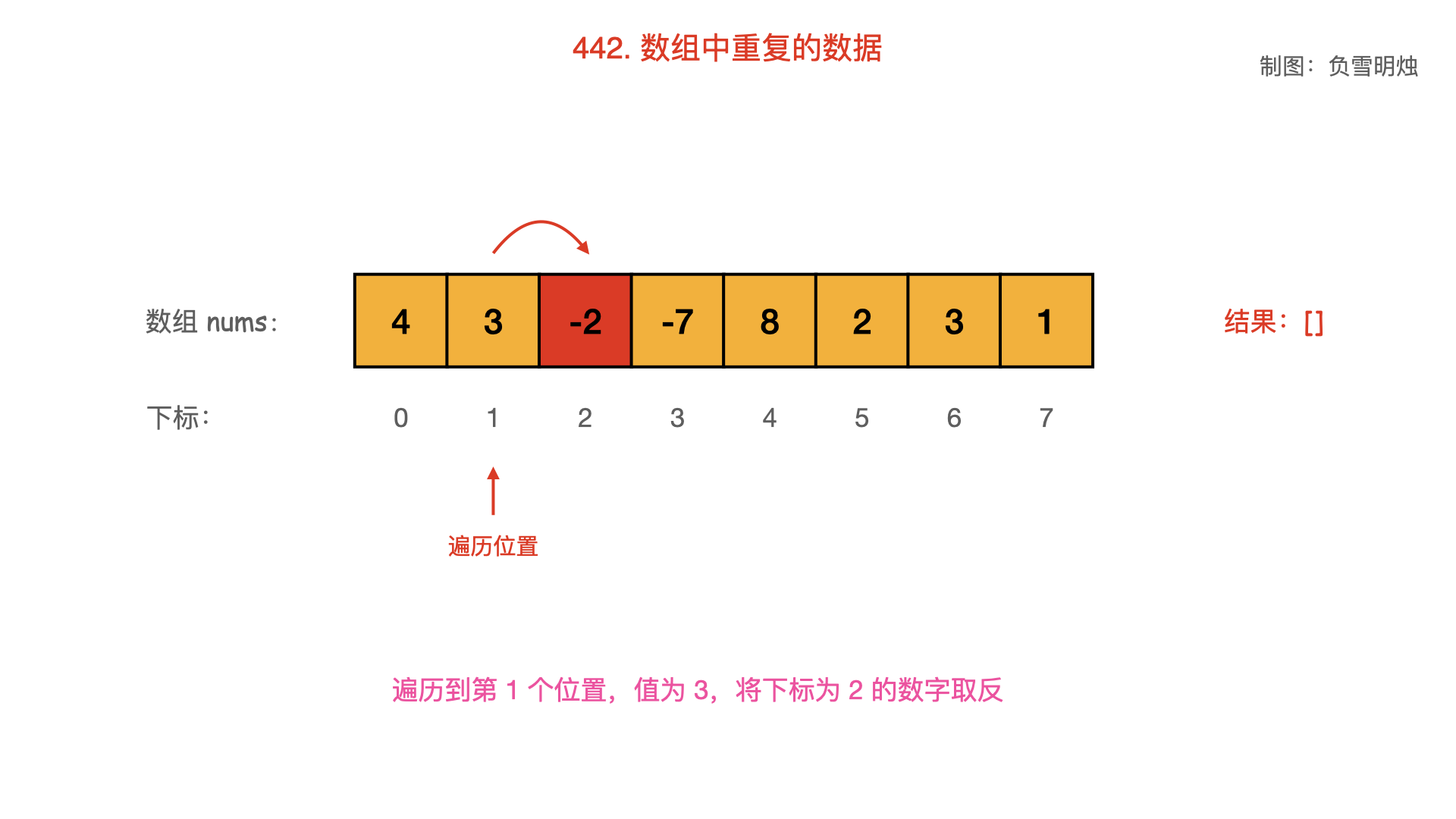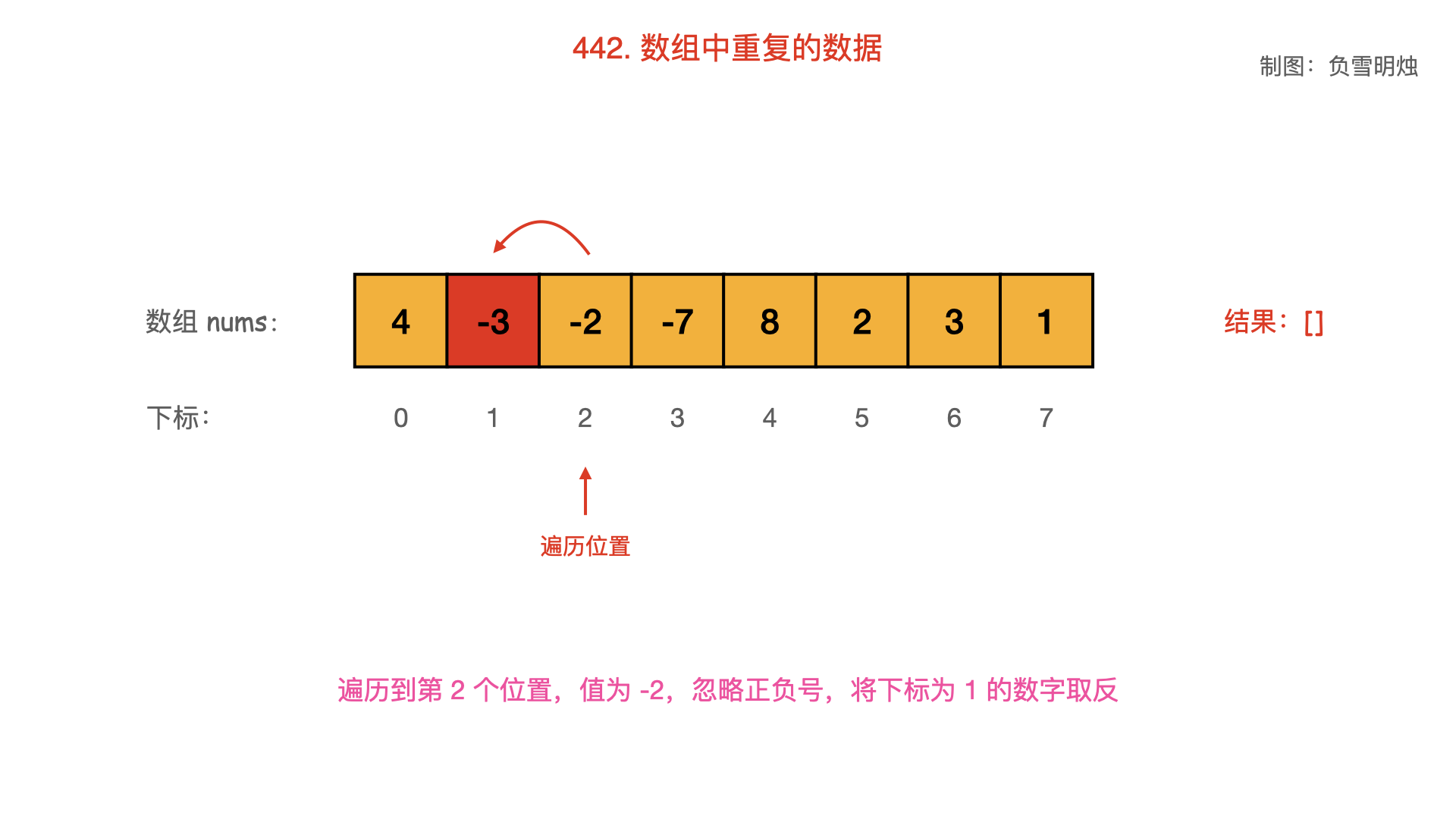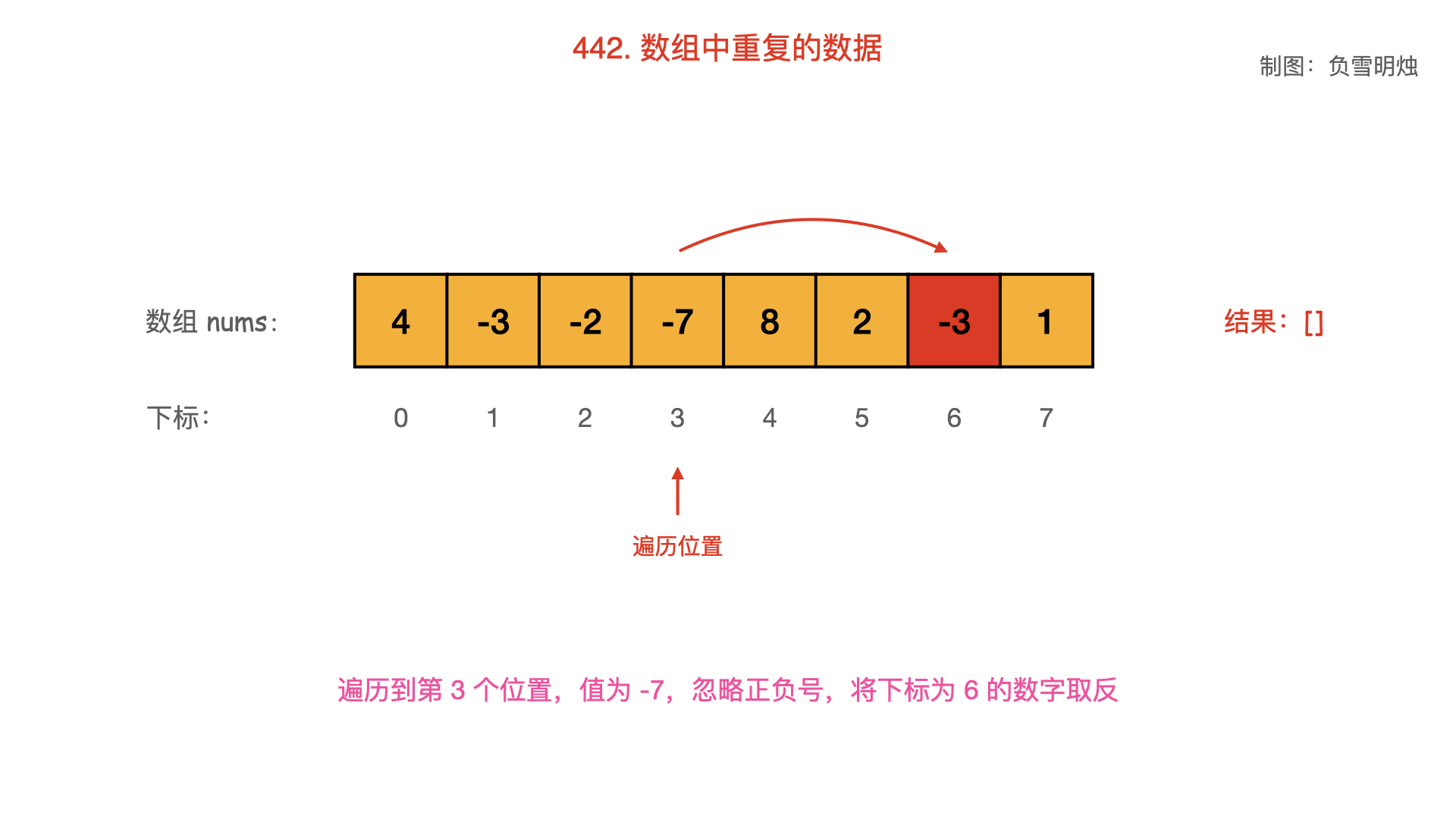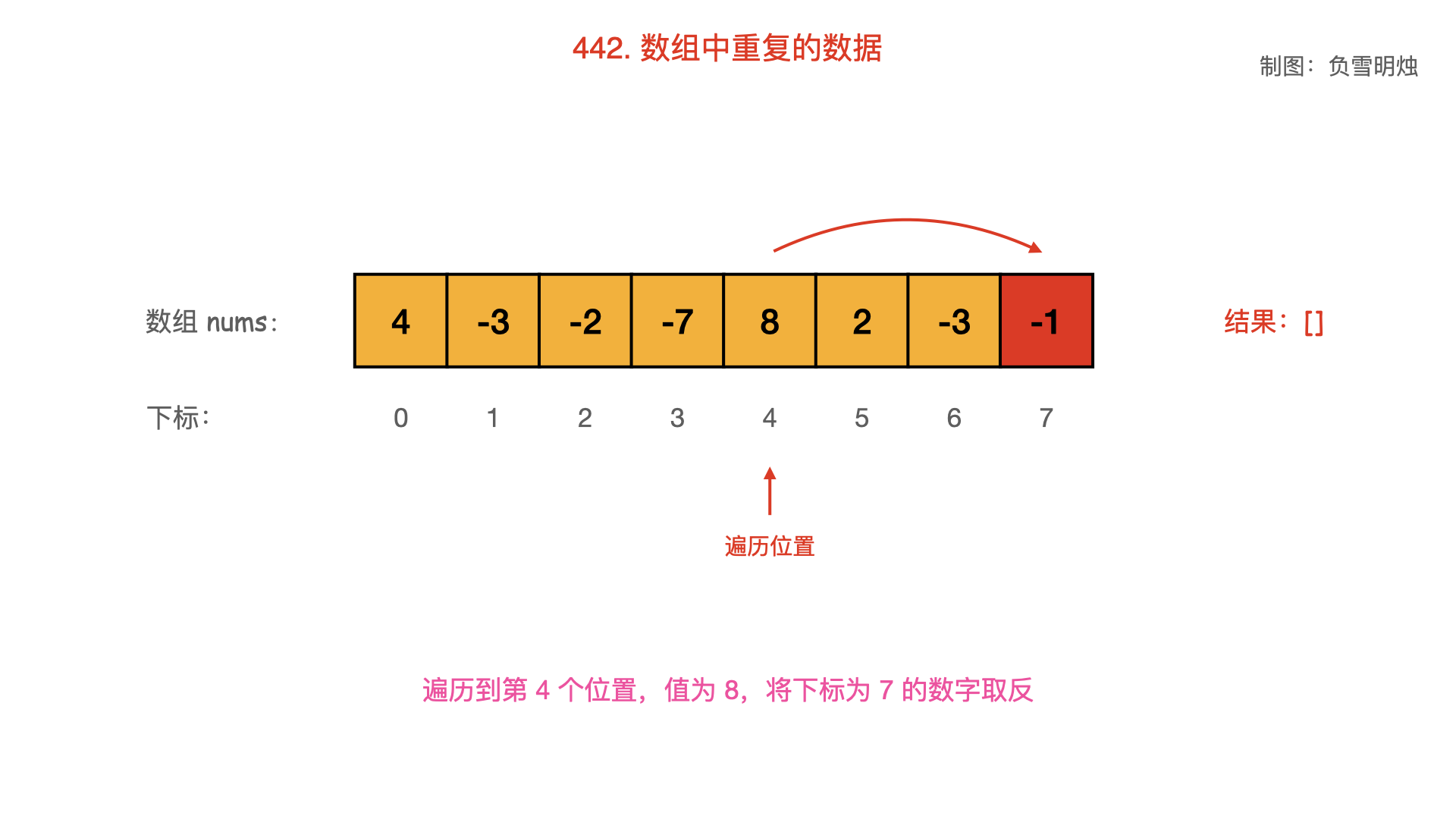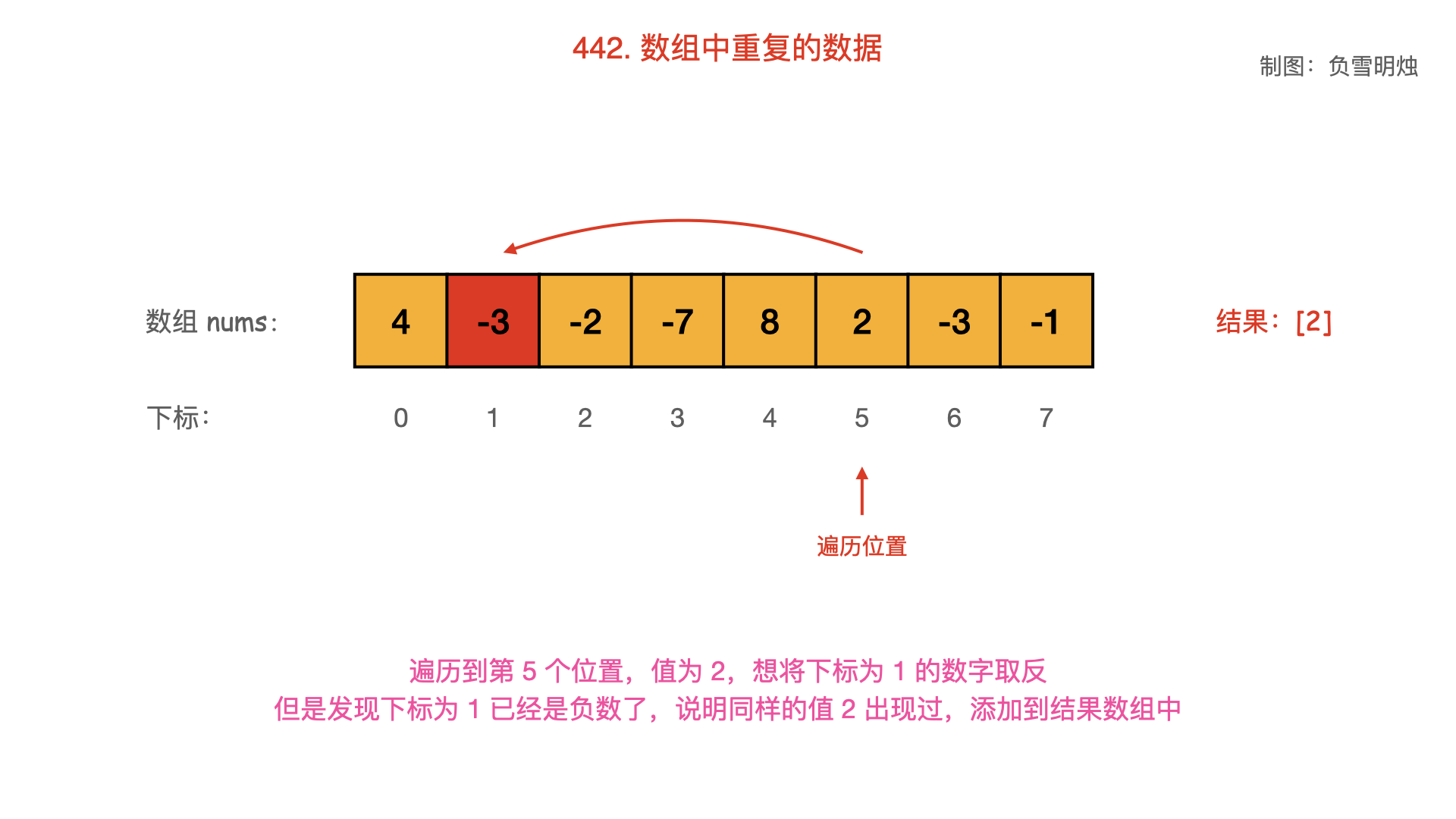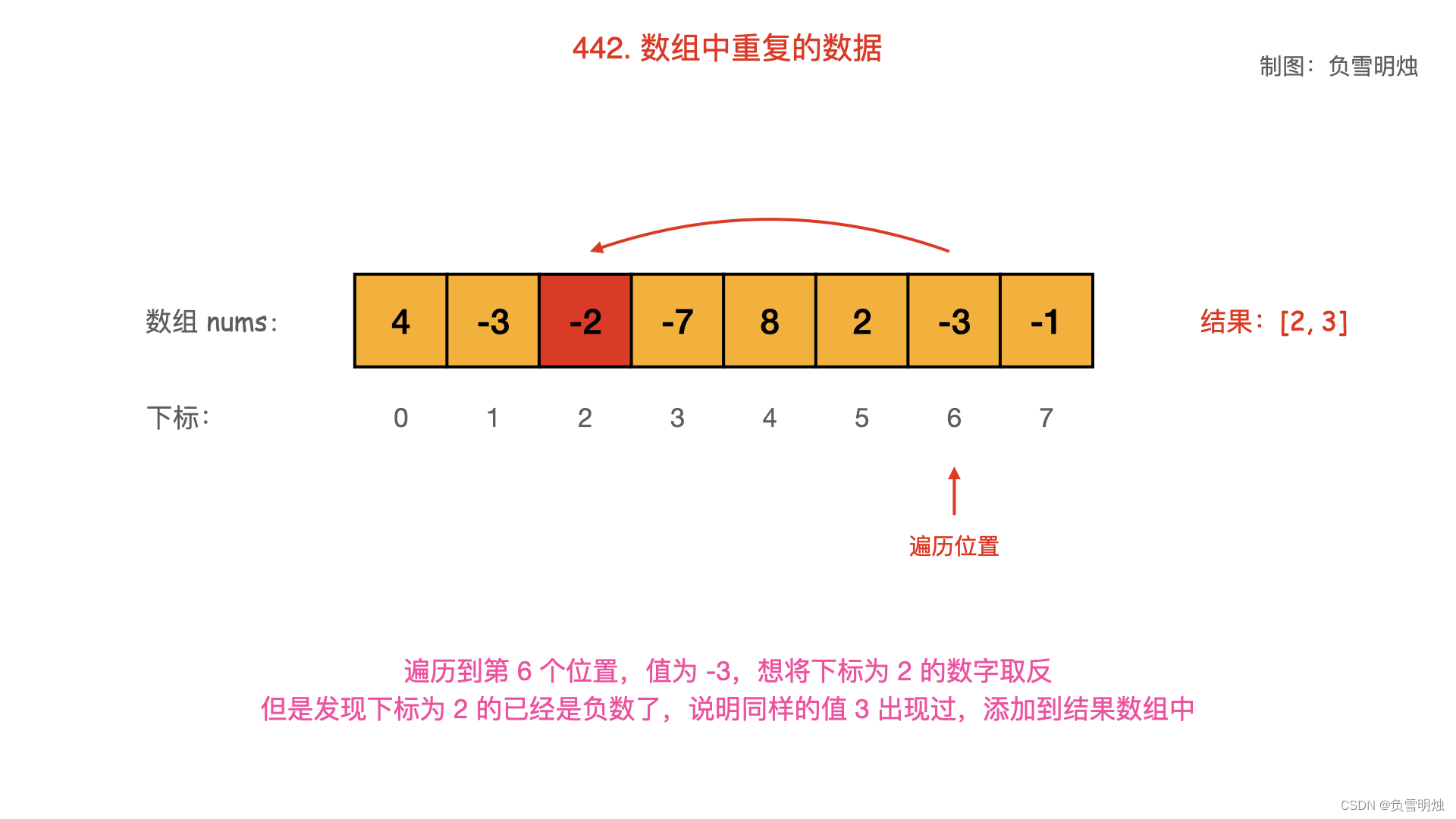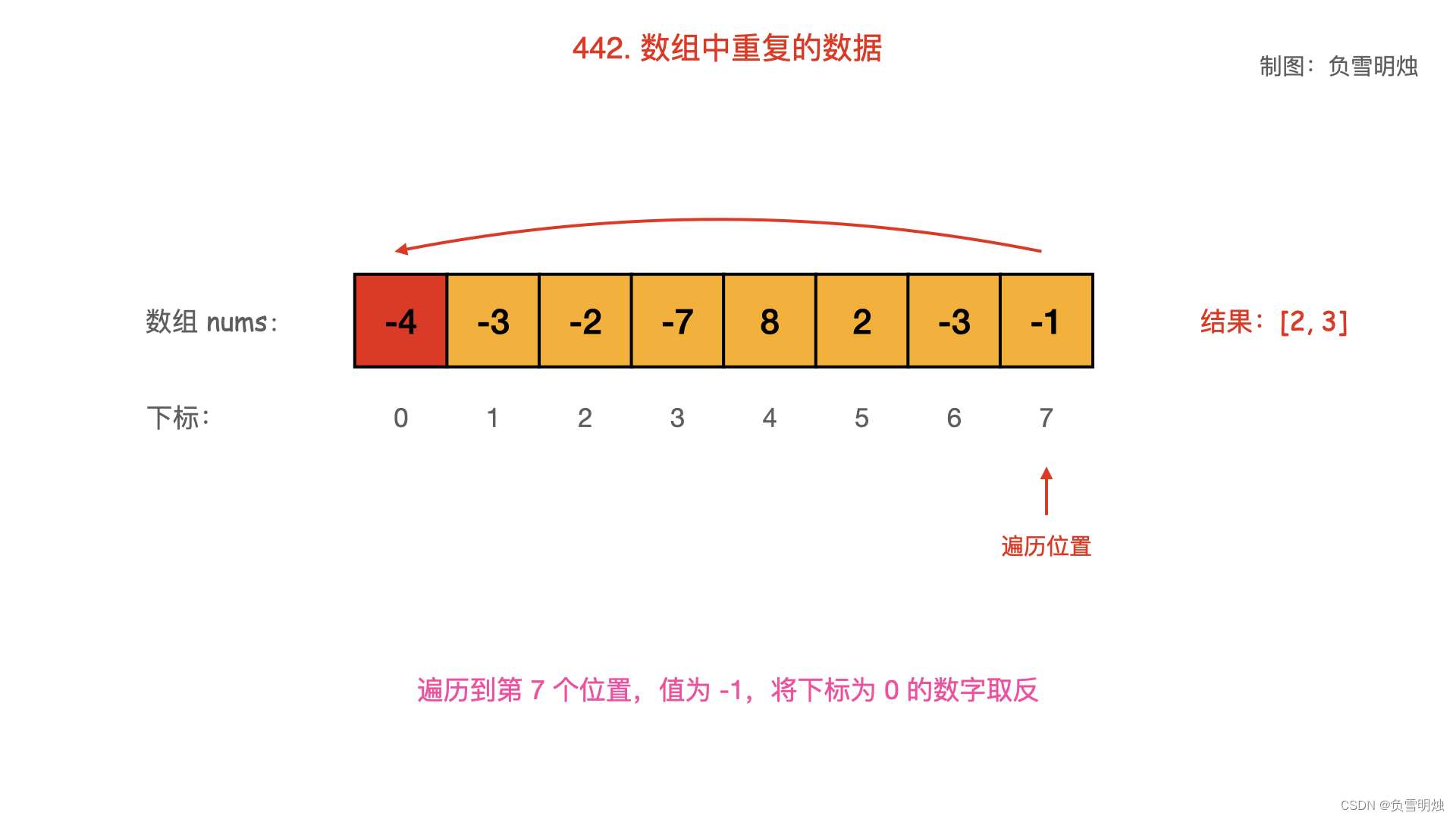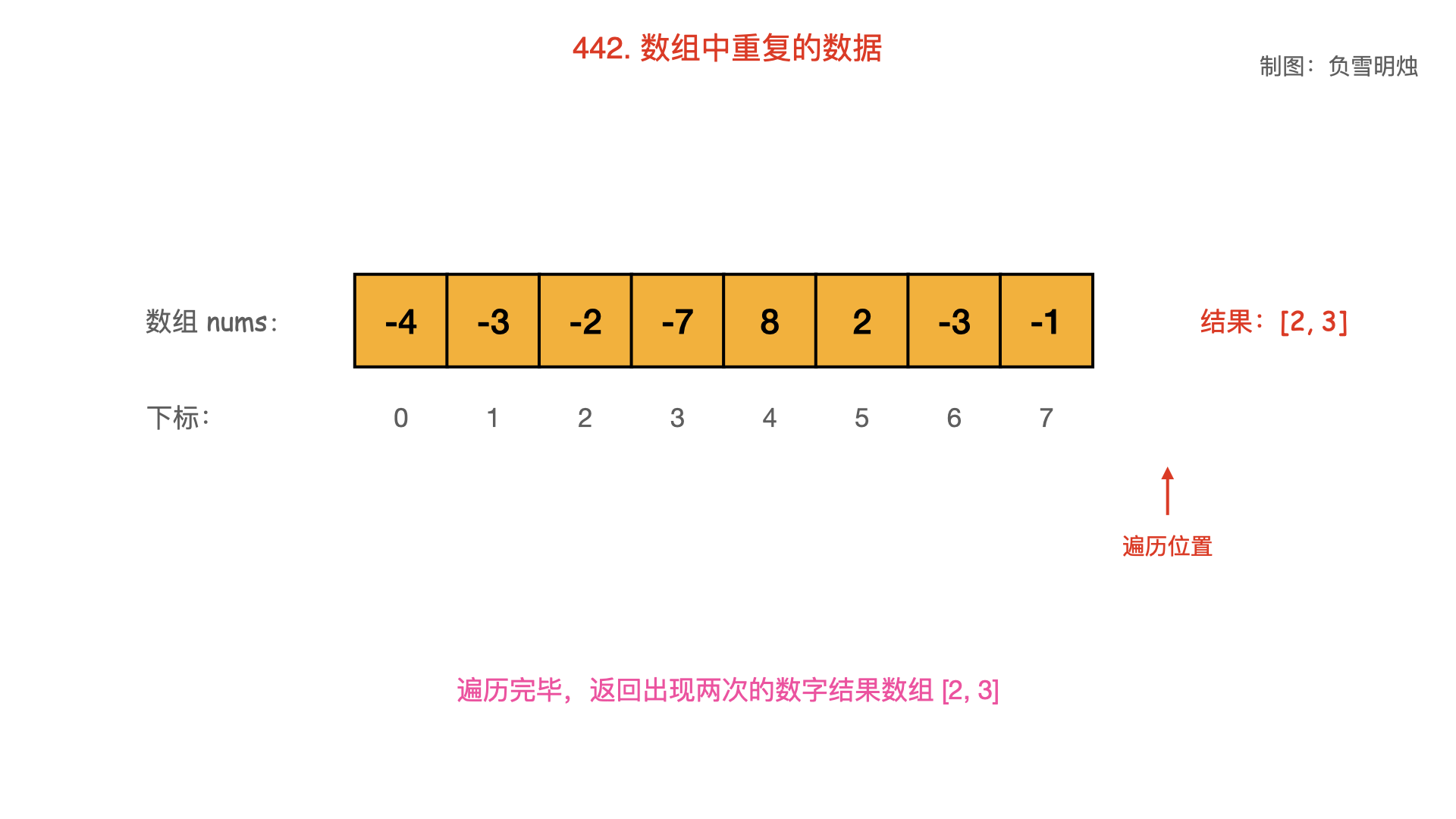Python 语言的代码如下：

class Solution(object):
def findDuplicates(self, nums):
ans = []
for num in nums:
if nums[abs(num) - 1] < 0:
ans.append(abs(num))
nums[abs(num) - 1] *= - 1
return ans


C++ 语言的代码如下：

class Solution {
public:
vector<int> findDuplicates(vector<int>& nums) {
const int N = nums.size();
vector<int> res;
for (int i = 0; i < N; i++) {
if (nums[abs(nums[i]) - 1] < 0)
res.push_back(abs(nums[i]));
nums[abs(nums[i]) - 1] *= -1;
}
return res;
}
};


Java 语言的代码如下：

class Solution {
public List<Integer> findDuplicates(int[] nums) {
List<Integer> res = new ArrayList<>();
for (int num : nums) {
if (nums[Math.abs(num) - 1] < 0) {
} else {
nums[Math.abs(num) - 1] *= -1;
}
}
return res;
}
}


#### # 方法二：增加偏移量

• 从起始位置进行遍历，每次将下标的数字
• 遍历到值 超过 ，需要将其 恢复原值；
• 若发现下标 的数字已经超过 ，说明之前出现过同样的数字 ，即找到了重复数字；

Python 语言的代码如下：

class Solution(object):
def findDuplicates(self, nums):
res = []
for num in nums:
if nums[(num % 100000) - 1] > 100000:
res.append(num % 100000)
else:
nums[(num % 100000) - 1] += 100000
return res


C++ 语言的代码如下：

class Solution {
public:
vector<int> findDuplicates(vector<int>& nums) {
vector<int> res;
for (int num : nums) {
if (nums[(num % 100000) - 1] > 100000) {
res.push_back(num % 100000);
}
nums[(num % 100000) - 1] += 100000;
}
return res;
}
};


Java 语言的代码如下：

class Solution {
public List<Integer> findDuplicates(int[] nums) {
List<Integer> res = new ArrayList<>();
for (int num : nums) {
if (nums[(num % 100000) - 1] > 100000) {
} else {
nums[(num % 100000) - 1] += 100000;
}
}
return res;
}
}


• 时间复杂度：
• 空间复杂度：

## # 总结

1. 这个题是技巧题目，看懂了我的题解以后，以后遇到同样的题目，应该就会了。

## # 日期

2018 年 2 月 6 日 2018 年 12 月 5 日 —— 周三啦！ 2022 年 5 月 8 日 —— 五一调休后，本周末只有一天休息